Homework Help Question & Answers

# and z2 = 1 1 + 3i 3-i a) Given that zı = find z such...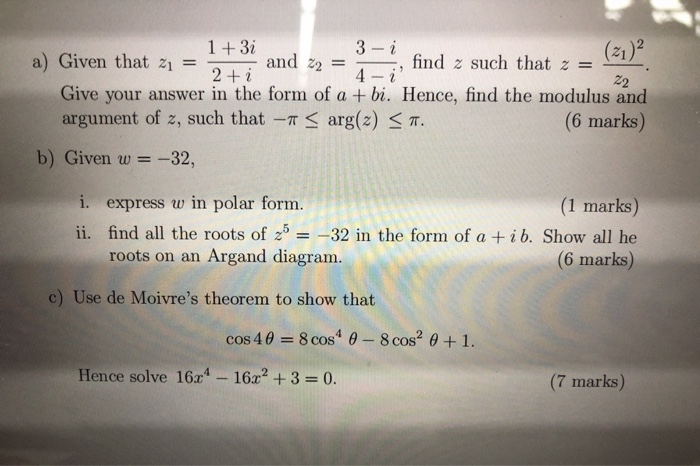and z2 = 1 1 + 3i 3-i a) Given that zı = find z such that z = 2 + i 4- ¿ 22 Give your answer in the form of a + bi. Hence, find the modulus and argument of z, such that -- < arg(2) < 7. (6 marks) b) Given w = = -32, i. express w in polar form. (1 marks) ii. find all the roots of 2b = -32 in the form of a +ib. Show all he roots on an Argand diagram. (6 marks) c) Use de Moivre's theorem to show that cos 40 = 8 cos 0 - 8 cos0 +1. Hence solve 163-16x2 + 3 = 0. (7 marks)

#### Homework Answers

Answer #1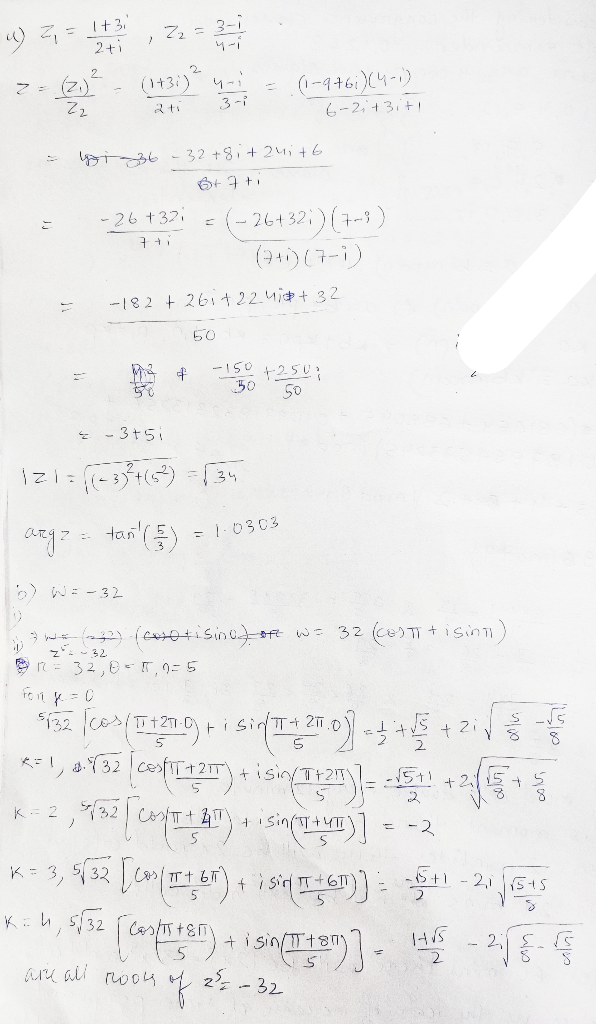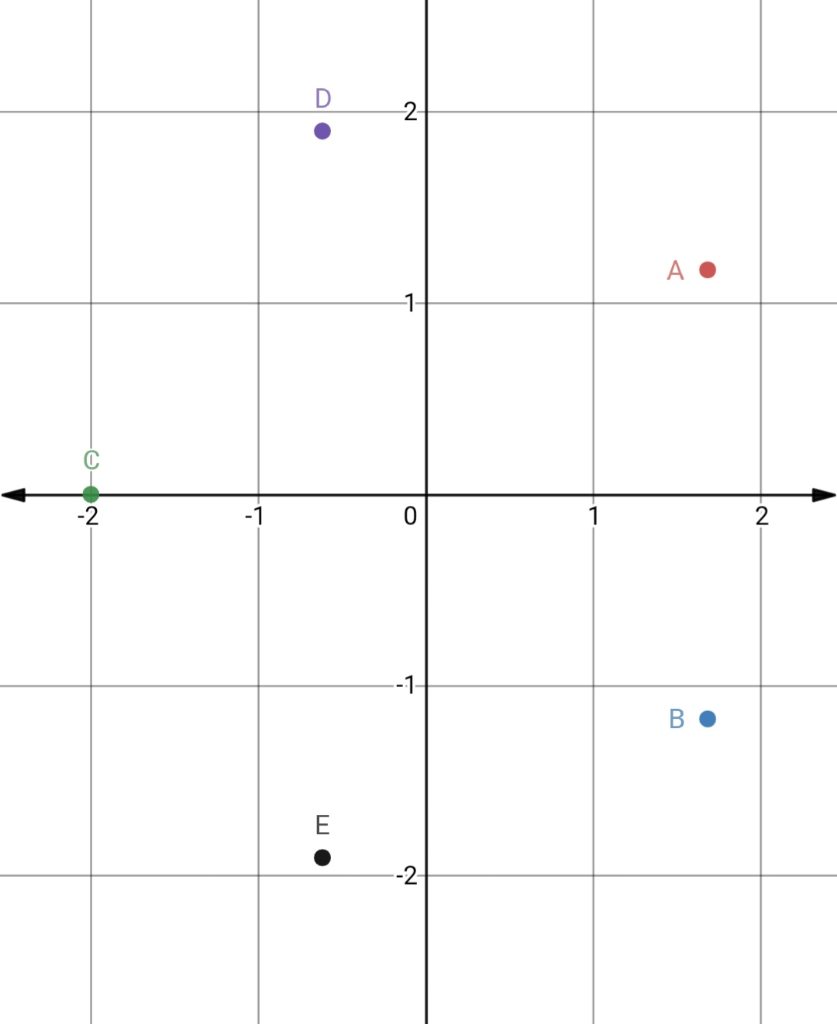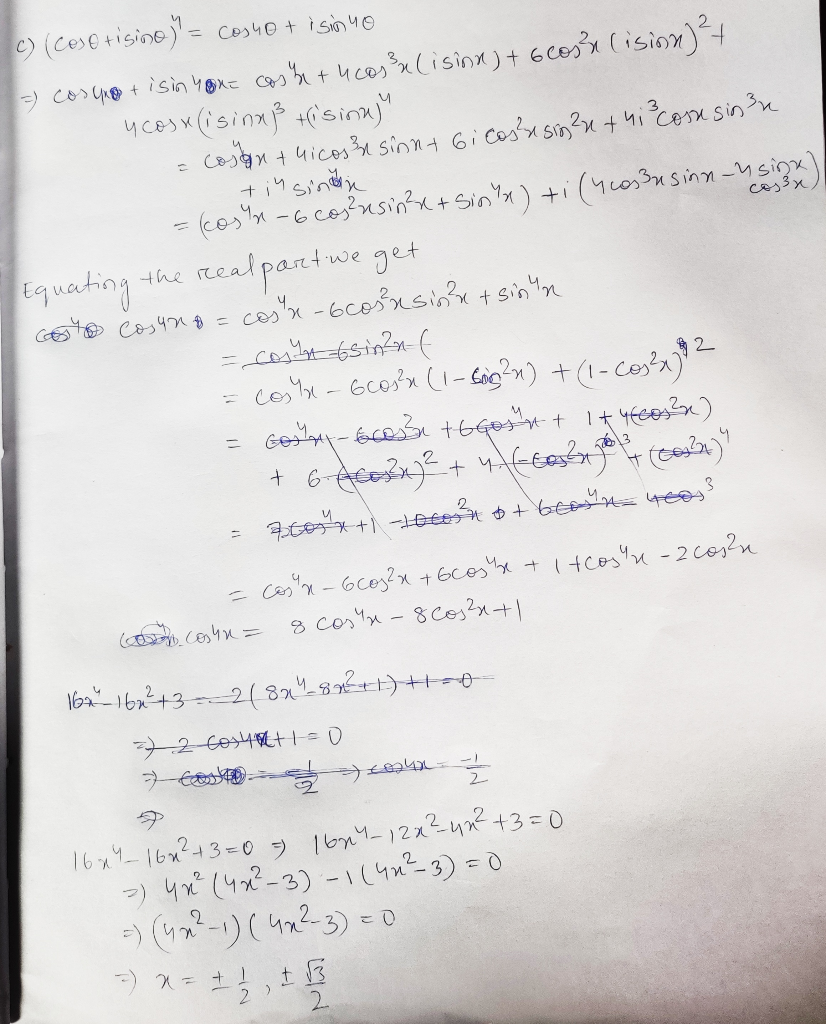Know the answer?
Your Answer:

#### Post as a guest

Your Name:

What's your source?

#### Earn Coin

Coins can be redeemed for fabulous gifts.

Not the answer you're looking for? Ask your own homework help question. Our experts will answer your question WITHIN MINUTES for Free.
Similar Homework Help Questions
• ### step by step solution thanks alot BurAkEsEN. NAME: Q1. (30pts) Solve the quadratic equation z2(6 3i)z...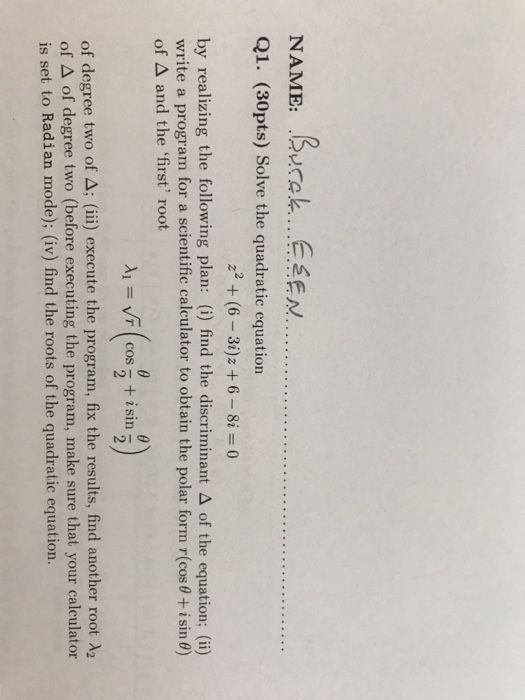step by step solution thanks alot BurAkEsEN. NAME: Q1. (30pts) Solve the quadratic equation z2(6 3i)z +6-8i 0 by realizing the following plan: (i) find the discriminant A of the equation; (ii) write a program for a scientific calculator to obtain the polar form r(cos0+ i sin 0) of A and the 'first' root + isin 2 COS of degree two of A; (iii) execute the program, fix the results, find another root A2 of A of degree two (before...

• ### NAME Q1. (30pts) Solve the quadratic equation z2-(3+3i)z +6+2i = 0 by realizing the following plan:...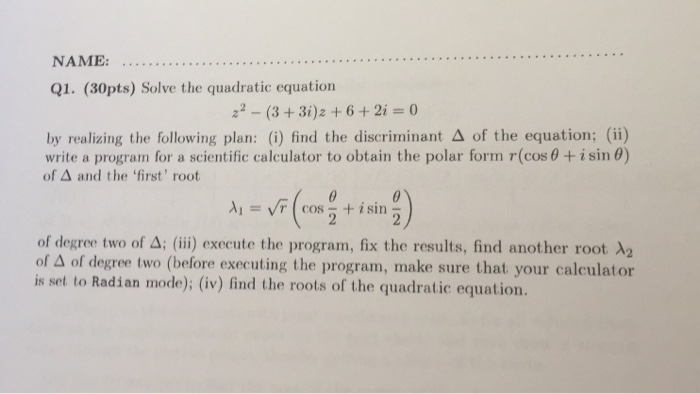NAME Q1. (30pts) Solve the quadratic equation z2-(3+3i)z +6+2i = 0 by realizing the following plan: (i) find the discriminant A of the equation; (ii) write a program for a scientific calculator to obtain the polar form r(cos 0 + i sin 0) of A and the 'first' root + isin COS 2 of degree two of A; (iii) execute the program, fix the results, find another root A2 of A of degree two (before executing the program, make sure...

• ### Find the following: z2+9 e) lim 23į 2-3i z2+i f) lim 2-i 24-1 Write given numbers...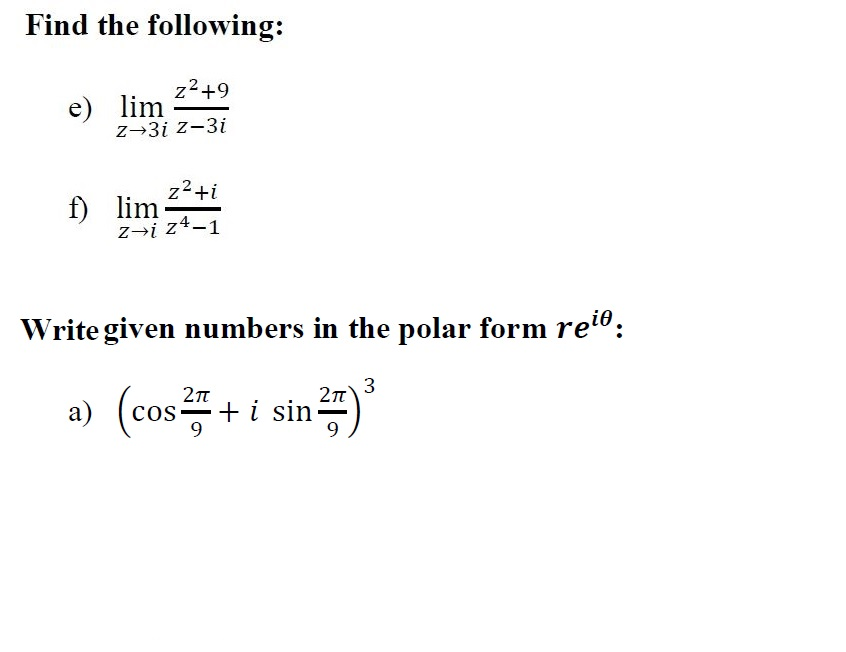Find the following: z2+9 e) lim 23į 2-3i z2+i f) lim 2-i 24-1 Write given numbers in the polar form reio: 3 a) (cos 29 + i sin 27)

• ### detailed solution for this one ????? 11. (a) Gi) If w=z+z-' prove that (i) z2 +...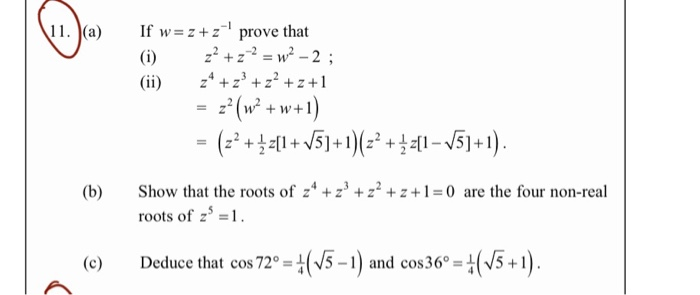detailed solution for this one ????? 11. (a) Gi) If w=z+z-' prove that (i) z2 + z 2 = w2 -2 ; 24 +2° + z²+z+1 = z2 (W2 + w+1) = (z? +[1+V5]+1)(22 +[1–V5]+1). (b) Show that the roots of 24 +2+z2+z+1=0 are the four non-real roots of z' =1. (c) Deduce that cos 72° = +(15 – 1) and cos 36° = (15+1).

• ### I. Given Z-2- i and Z2-1 2i. Find the following and express your answer in the...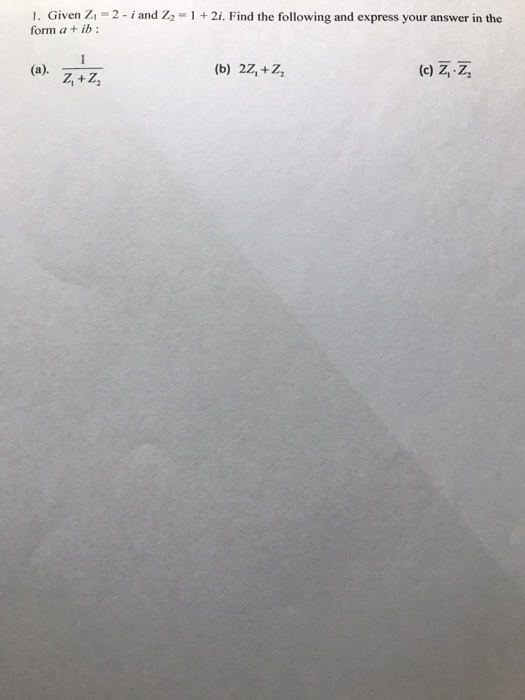I. Given Z-2- i and Z2-1 2i. Find the following and express your answer in the form a+ ib (c) Z, Z, (b) 22, +Z, (a).

• ### Find R and angle. Z1 =8+3i, Z2 =2+3i, Z3 =9-((2)^1/2 )i. (vi) z = TEM (vii)...Find R and angle. Z1 =8+3i, Z2 =2+3i, Z3 =9-((2)^1/2 )i. (vi) z = TEM (vii) 2 = 22 + 231

• ### 2iz-1 If f(2) = 27251 2-3i a) Find and simplify f'(z) b) Find f'(1 + i)...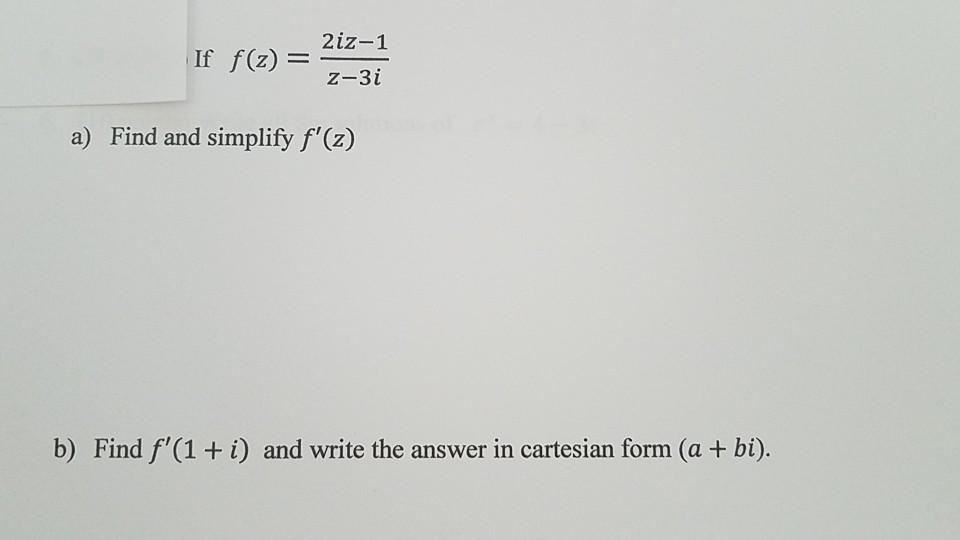2iz-1 If f(2) = 27251 2-3i a) Find and simplify f'(z) b) Find f'(1 + i) and write the answer in cartesian form (a + bi).

• ### For the complex number given as: z = a + bi / c+di where i =...

For the complex number given as: z = a + bi / c+di where i = √−1 is the imaginary unit. The parameters are defined as a = √2, b = 0, c = 0.5 and d = −0.5. (a) Find the real and the imaginary parts of z, and then draw the Argand dia- gram. (Hint: Use the conjugate of the denominator.) 2.5 (b) Based on the Argand diagram, find the distance r of the complex number z from...

• ### 1 2 NAME Q1. (30pts) Solve the quadratic equation z2-(3+3i)z +6+2i = 0 by realizing the...1 2 NAME Q1. (30pts) Solve the quadratic equation z2-(3+3i)z +6+2i = 0 by realizing the following plan: (i) find the discriminant A of the equation; (ii) write a program for a scientific calculator to obtain the polar form r(cos 0 + i sin 0) of A and the 'first' root + isin COS 2 of degree two of A; (iii) execute the program, fix the results, find another root A2 of A of degree two (before executing the program,...

• ### Find the complex numbers w and z which solve the system of equations (-1+i)w + (-2-3i)z...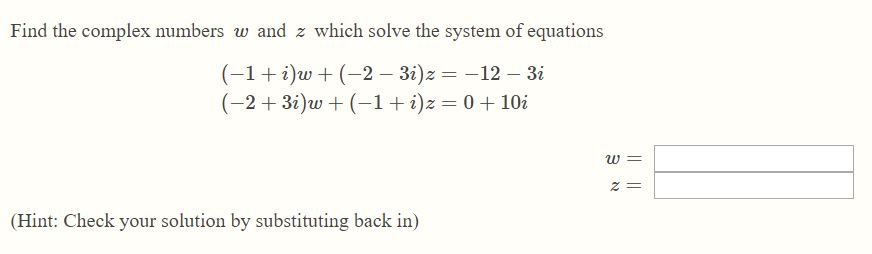Find the complex numbers w and z which solve the system of equations (-1+i)w + (-2-3i)z = -12 - 3i (-2+3i)w +(-1+i)z = 0 +10i (Hint: Check your solution by substituting back in)

Free Homework App

Scan Your Homework
to Get Instant Free Answers
Need Online Homework Help?

Get Answers For Free
Most questions answered within 3 hours.